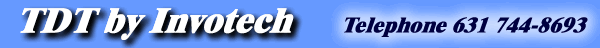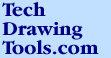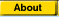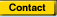TDT - Productivity solutions for tech illustrators.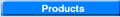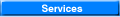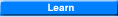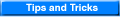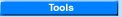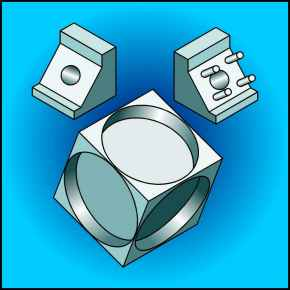Off Axis - Tangents - page 1 In this lesson you will develop a projection that is off axis and on a tangent to the isometric plane. Let me stress once more that a successful illustrator is be able to picture what an off angle object looks like before they begin to draw. Purchase our Tech Drawing Tools and work more efficiently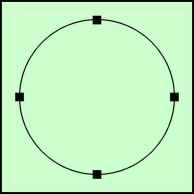Before we can begin you must create a set of master ellipses. Create a perfect circle by choosing the ellipse tool (F7) and while holding down the constrain key (ctrl) drag across the screen to about 2 inches (Watch the status line). Convert this to a curve (Ctrl+Q) to place 4 nodes around the circle. These nodes will be at the major and minor axis’s.Use the Transform Roll-up to apply to a duplicate the following:  5 degree ellipse = 10% vertical scale. 10 degree ellipse = 18% vertical scale. 15 degree ellipse = 26% vertical scale. 20 degree ellipse = 34% vertical scale. 30 degree ellipse = 50% vertical scale. Isometric ellipse (35 degree 16 minutes) = 57.4% vertical scale. 40 degree ellipse = 63% vertical scale. 45 degree ellipse = 70% vertical scale. 50 degree ellipse = 76% vertical scale. 55 degree ellipse = 79% vertical scale. 60 degree ellipse = 83% vertical scale. 65 degree ellipse = 88% vertical scale. 70 degree ellipse = 94% vertical scale. 80 degree ellipse = 97% vertical scale. 90 degree ellipse = the perfect circle. Note: Other ellipses can be created by extrapolating the above table.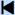Start 1 2 3 4 5 6 7 8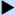Next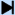End Learn More